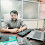# ATM Program in Python

2

Create ATM Program with all operation when user will enter correct pin code then it will process
proceed  to next screen with following menu option
1.   Press C for Credit
2.   Press D for Debit
3.   Press B for Check Balance
4:-  Press R for Repeat
5:-  Press E for exit
If Pin code will be incorrect then three attempt should be provide to user's to enter right pin code otherwise exit from System.
User can perform only three operation with one login session ,display complete log details after exit from System.
pin='1111'
flag=False
amt=5000
count=0
for i in range(1,4):
pin = input("enter pin code")
if pin=='1111':
flag=True
break
if flag:
while(True):
if count>3:
exit(0)
operation = input("""
press d for debit
press b for check balance
press e for exit and press
r for repeate operation""")
if(operation =='d'):
debit_amount = int(input("please enter the amount you want to debit"))
if(debit_amount<amt):
amt = amt-debit_amount
print("now your current balance is ",amt,"and you have debited rupees ",debit_amount)
count = count+1
print(count)
else:
print("no sufficient balance in your account")
if(operation =='c'):
credit_amount = int(input("please enter the amount you want to credit"))
amt = amt+credit_amount
print("now your current balance is ",amt,"and you have credited rupees ",credit_amount)
count = count+1
print(count)
if(operation =='b'):
print("your current balance is = ",amt)
count = count+1
print(count)
if(operation =='e'):
exit(0)
if(operation=='r'):
count=0
continue
else:
print("you have entered wrong pin code and you complete your attempt"
Tags

1.PIN=3108
b=75000
k=3
flag=False
count=1
log=""
while(k):
p=int(input("Enter PIN : "))
if(PIN==p):
flag=True
break

else:
print("Enter Correct PIN.....")
k-=1
else:
print("Attempt Completed.....")

while(flag and count<=3):
print("Welcome Shivam......")
print("for Credit, press C\ for Debit, press D")
print("for checkBALACE, press B\n for Exit, press E")
print("for Continue, press C")
s=input("what you have to do : ")
if(s=='d' or s=='D'):
putBALANCE=int(input("Enter ammount : "))
log += "DEBIT OPERATION \n"
if putBALANCE<=b:
b-=putBALANCE;
log += "DEBIT AMOUNT IS "+str(b)+" \n"
else:
log += "DEBIT AMOUNT IS Insufficient balance \n"
print("Insufficient balance")
count+=1
elif(s=='c' or s=='C'):
putBALANCE=int(input("Enter ammount : "));
log += "CREDIT OPERATION, Credit amount "+str(putBALANCE)+"\n"
b+=putBALANCE;
count+=1
log += "TOTAL AMOUNT IS "+str(b)+"\n"
elif(s=='b' or s=='B'):
print("Your curret balance is : ",b)
log += "TOTAL BALANCE IS "+str(b)+"\n"
count+=1
elif(s=='e' or s=='E'):
flag=False
elif(s=='c' or s=='C'):
continue;
else:
if c>3:
print("You can only perform three operation")
print(log)

2.pin='1111'
flag=False

def processATM(flag):
count=0
amt=5000
if flag:
while(True):
if count>3:
exit(0)
operation = input("""
press d for debit
press b for check balance
press e for exit and press
r for repeate operation\n:""")

match operation:
case 'd':
debit_amount = int(input("please enter the amount you want to debit:"))
if(debit_amount<amt):
amt = amt-debit_amount
print("now your current balance is ",amt,"and you have debited rupees ",debit_amount)
count = count+1
print(count)
else:
print("no sufficient balance in your account")
case 'c':
credit_amount = int(input("please enter the amount you want to credit:"))
amt = amt+credit_amount
print("now your current balance is ",amt,"and you have credited rupees ",credit_amount)
count = count+1
print(count)
case 'b':
print("your current balance is = ",amt)
count = count+1
print(count)
case "e":
exit(0)
case "r":
count=0
continue

else:
print("you have entered wrong pin code and you complete your attempt")

for i in range(1,4):
pin = input("enter pin code:")
if pin=='1111':
flag=True
break